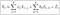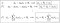# A Complete Introduction To Time Series Analysis (with R):: Gaussian Time Series

In the last two articles, we saw a number of methods to independently estimate AR(p) and MA(q) coefficients, namely the Yule-Walker method, Burg’s Algorithm, and the Innovations Algorithm, as well as the Hannan-Risennan Algorithm, to jointly estimate ARMA(p,q) coefficients, by making use of initialized AR(p) and MA(q) coefficients with the previous algorithms. We also mentioned, that these methods, as sophisticated as they are, tend to perform quite poorly when it comes to dealing with real datasets, as it’s easy to misspecify the true model. Therefore, we would like to yer introduce another assumption: normality of the observations. …

# A Complete Introduction To Time Series Analysis (with R):: Estimation of ARMA(p,q) Coefficients (Part II)The ARMA(p,q) model implies that X_{t} can be expressed in the form above.

In the last article, we learned about two algorithms to estimate the AR(p) process coefficients: the Yale-Walker equations method, and Burg’s algorithm. In this article, we will now see a very simple way to determine the MA(q) process coefficients, and a first approach to estimate the ARMA(p,q), jointly. Let’s see how this works:

## Estimation of MA(q) (Innovations)

As you may guess by the title, the way to estimate the MA(q) coefficients is… the Innovations Algorithm we saw before. Recall that the MA(q) process can be written as

# A Complete Introduction To Time Series Analysis (with R):: Estimation of ARMA(p,q) Coefficients (Part I)

In the last article, we discussed the extension of the Innovations algorithm for the more general ARMA(p,q) process, which allowed us to make predictionsf for arbitrary number of timesteps in the future. However, we still haven’t seen how to estimate the actual ARMA(p,q) model coefficients. In this article, we will see two algorithms for estimating AR(p) coefficients, and in the next article, we will see how to estimate MA(q) and start taking a look into jointly estimating ARMA(p,q) coefficients. Let’s jump right into it!

## Estimation of AR(p) :: Yale-Walker

In real world problems, the ACVF is the easiest thing to estimate using the sample data. …

# A Complete Introduction To Time Series Analysis (with R):: Prediction III: Forecasting with ARMA(p,q) models

We have come a long way from first exploring the idea of models with way too little or too much dependence, to the structured ARMA(p,q) models that aim to balance this by taking into account not only dependence between observations, but between their random noise at different timesteps. In the “Prediction II: Forecasting” section, we studied the best linear predictor along with two algorithms to help us find the BLP coefficients and make predictions: the Durbin-Levinson algorithm and the Innovations algorithm. In this article, we will see how to extend these ideas to produce predictions for ARMA(p,q) models. Before starting, I strongly suggest you review the first article on the Innovations algorithm since this one builds directly on that one. …

# A Complete Introduction To Time Series Analysis (with R):: The ACF and PACF functions

In the last article, we discussed the stationarity, causality, and invertibility properties of ARMA(p,q) process, along with the conditions required to ensure these, and how to verify them. In this article, we will see how these properties, in particular, stationarity and causality greatly simplify our task of finding the ACVF, ACF, and PACF.

## ARMA(p,q) as a Linear Process

Recall from this article that a linear process is no more than a stationary time series which has the representation

# A Complete Introduction To Time Series Analysis (with R):: ARMA processes (Part II)The coefficients of the causal representation of an ARMA(p,q) process are given by the recurrence relation above.

In the last article, we saw that a general ARMA(p,q) process can be written, with the help of the autoregressive and moving-average operators as

# A Complete Introduction To Time Series Analysis (with R):: ARMA processes (Part I)

Perhaps one of the most famous and best-studied approaches to working with time series, still widely used today is the ARMA(p,q) models and its derivatives. As you can guess, these essentially introduce a generalization of the AR(1) and MA(1) processes that we have previously seen. Before we start, let’s introduce some useful operators that will allow us to simplify our notation.

# A Complete Introduction To Time Series Analysis (with R):: Innovations Algorithm

In the last article, we studied in depth the famous Durbin-Levinson algorithm, which allowed us to recursively compute the coefficients of the best linear predictor given by

# A Complete Introduction To Time Series Analysis (with R):: Durbin-Levinson Algorithm

In the last article, we saw how we could find the form of the best linear predictor of X_{n+h} using all previous observations up to n has the form